ESAP达摩院 思想有多远，我们就能走多远

# ESAP高级教程|动态换算的奥义

## 课题引出

• 购入一批铝型材，仓库论米，生产切割成不同的长度论根，供应商结算论吨。

• 购入一批钢板，仓库论平方，生产切割论块，供应商结算论吨。

• 购入一批塑料膜，仓库论片，供应商结算论kg，并且四舍五入保留1位小数……

## 课题升级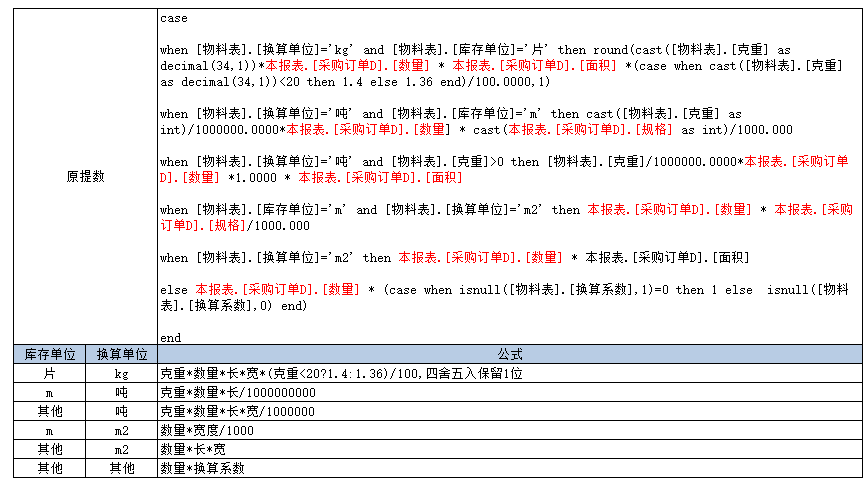## 方案评比

• 视图换算，这种换算实际就是：数量*常量，其中常量部分通过视图算出，然后匹配本表料号进行计算。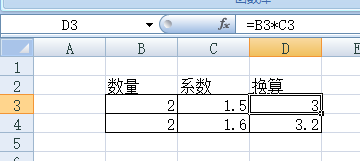• 使用EXCEL公式，这个是ES唯一原生支持的方案，从公式表提数填充到本表，类似：`=_克重*_数量*_长*_宽/1000000`，其中`_克重，_数量，_长，_宽`是定义的名称，代表某个字段区域。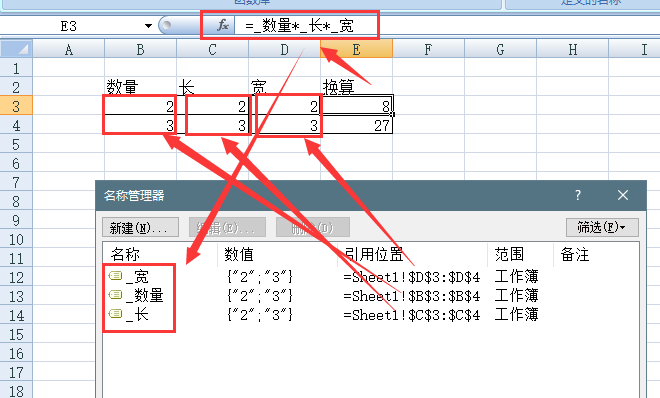• 使用sql函数，这个是NX原生支持的方案，将换算逻辑封装成一个sql函数，提数时无脑代入变量。
``````create function fGetAuxQty(
@qty decimal(34,3)=0   --数量
,@kz decimal(34,1)=0   --克重
,@gg varchar(128)=''  --规格，例如1000*500或800
--长=left(@gg,charindex('*',@gg+'*')-1),宽=right(@gg,len(@gg)-charindex('*',@gg+'*'))
,@dw varchar(128)=''  --库存单位
,@hs varchar(128)=''  --换算单位
,@hsxs varchar(128)=0  --换算系数
)
returns decimal(36,6)
as
begin
return
case
when @dw='片' and @hs='kg'
then round(@kz*@qty*cast(left(@gg,charindex('*',@gg+'*')-1)as int)*cast(right(@gg,len(@gg)-charindex('*',@gg+'*'))as int)*(case when @kz<20 then 1.4 else 1.36 end)/100000000.0000,1)
when @dw='m' and @hs='吨'
then @kz*@qty*cast(left(@gg,charindex('*',@gg+'*')-1)as int)/1000000000.000000
when @hs='吨' and @kz>0
then @kz*@qty*cast(left(@gg,charindex('*',@gg+'*')-1)as int)*cast(right(@gg,len(@gg)-charindex('*',@gg+'*'))as int)/1000000000000.000000
when @dw='m' and @hs='m2'
then @qty*cast(left(@gg,charindex('*',@gg+'*')-1)as int)/1000000.000000
when @hs='m2'
then @qty*cast(left(@gg,charindex('*',@gg+'*')-1)as int)*cast(right(@gg,len(@gg)-charindex('*',@gg+'*'))as int)/1000000.000000
else
@qty*(case when isnull(@hsxs,1)=0 then 1 else isnull(@hsxs,1) end)
end
end
``````

## 方案落地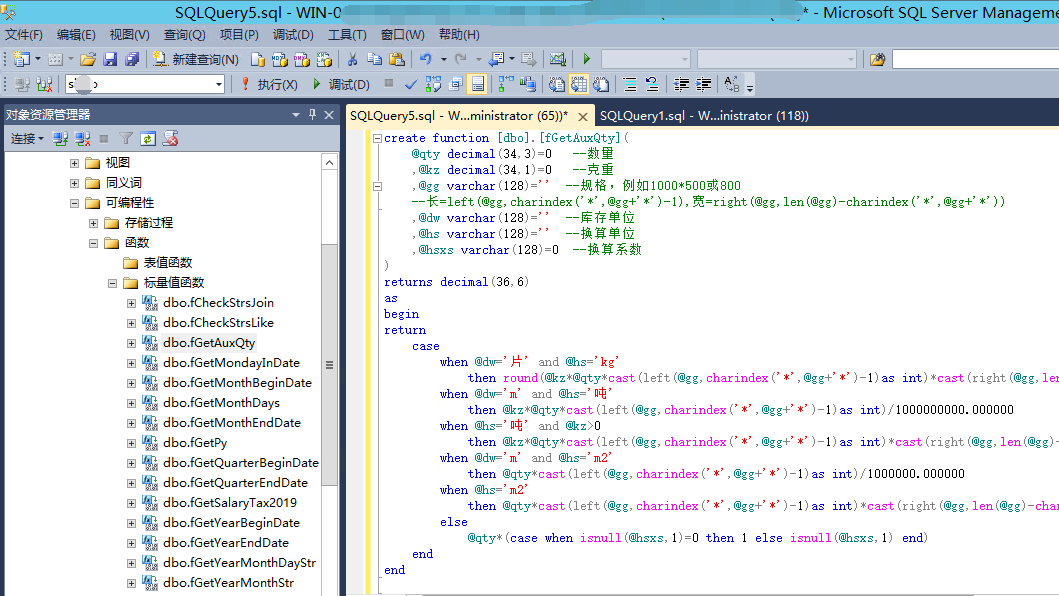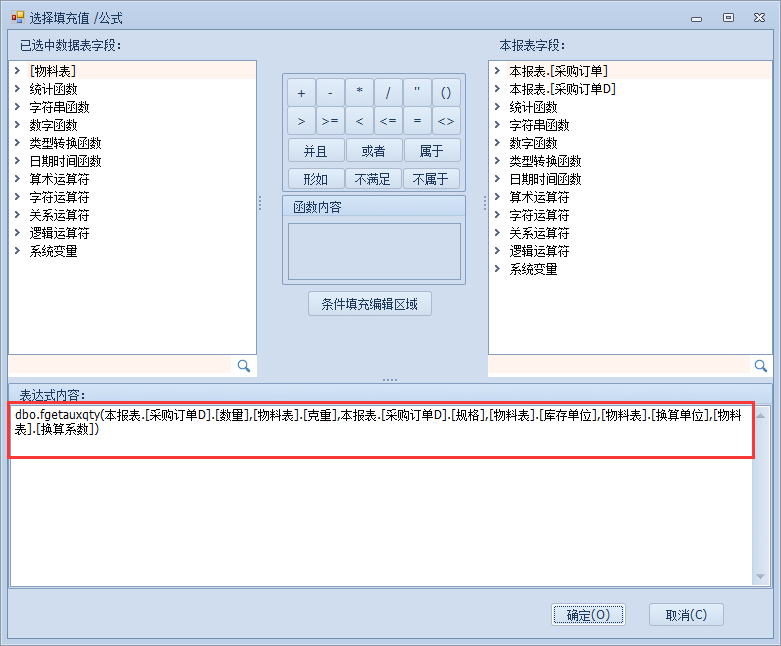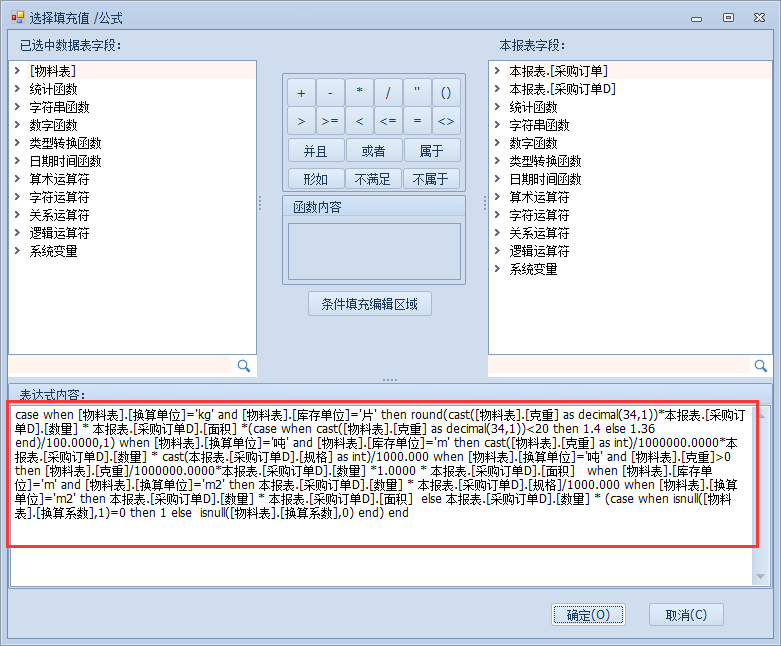## 最终效果示意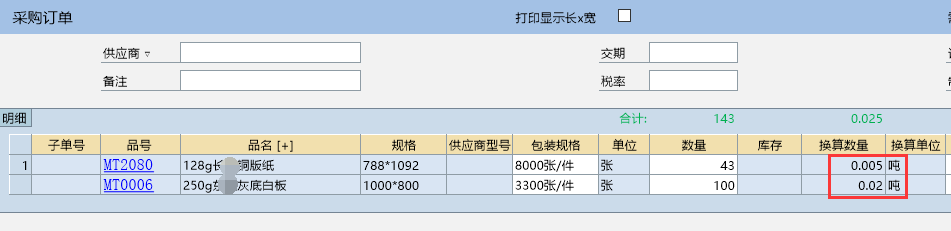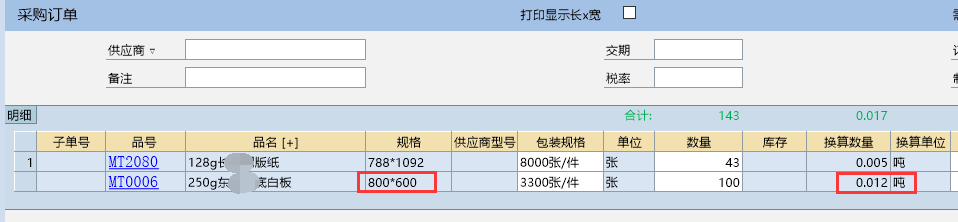## 小结

• 封装sql自定义函数一劳永逸；

• 万能提数中直接调用sql函数，无辅助字段，随叫随到。

• 感谢NX杨工提供的技术支持和的如此灵活的快开平台，并预祝筒子们元旦快乐~！

2019-12-31